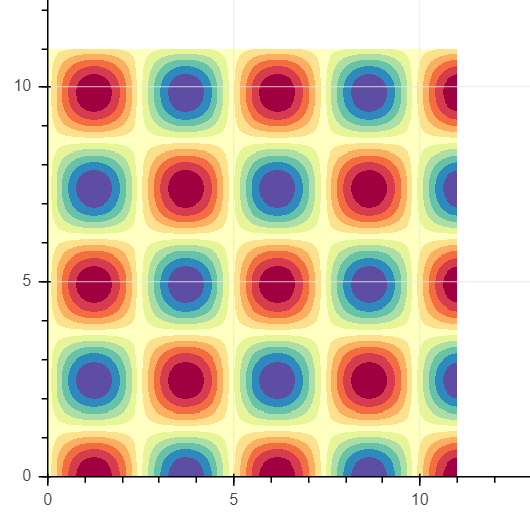# How can grid plot in Bokeh library be created with Python?

Bokeh is a Python package that helps in data visualization. It is an open source project. Bokeh renders its plot using HTML and JavaScript. This indicates that it is useful while working with web-based dashboards.

Bokeh converts the data source into a JSON file. This file is used as an input to BokehJS, which is a JavaScript library. This BokehJS is written in TypeScript that helps render visualization on modern browsers.

Dependencies of Bokeh −

Numpy
Pillow
Jinja2
Packaging
Pyyaml
Six
Python−dateutil

Installation of Bokeh on Windows command prompt

pip3 install bokeh


Installation of Bokeh on Anaconda prompt

conda install bokeh

## Example

import numpy as np

from bokeh.plotting import figure, output_file, show

N = 420
x = np.linspace(0, 14, N)
y = np.linspace(0, 14, N)
x1, y1 = np.meshgrid(x, y)
d = np.sin(x1)*np.cos(y1)

p = figure(tooltips=[("x", "$x"), ("y", "$y"), ("value", "@image")])

p.image(image=[d], x=0, y=0, dw=11, dh=11, palette="Spectral11", level="image")
p.grid.grid_line_width = 0.6

output_file("gridplot.html", title="grid plot example")

show(p)

## Output## Explanation

• The required packages are imported, and aliased.

• The figure function is called along with plot width and height.

• The data is defined using NumPy library.

• The ‘output_file’ function is called to mention the name of the html file that will be generated.

• The ‘image’ function present in Bokeh is called, along with data.

• The ‘show’ function is used to display the plot.